### Oh, Curvey!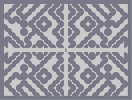Hover over the thumbnail for a full-size version.

Author fusion author:fusion n-art rated 2005-12-28 3 by 7 people. \$Oh, Curvey!#fusion#none#00;<0:<0;1<0;1<0;=0;<000;=:<0:<:<000;=;=0;=:<0;=0;=00:<:<0;=;=0;=00:<=;<:<000:<:0=;=00:<0;<:;=:<1<;<0:<0;=0;<;1;=:<=0;=:=:=;<;0<;<:=:=:<0:0;=0;<0;=:=0:=:<0;<0:<0;=0;=:1=00;0<00:1=:<0:<=0;=;<:<0;<0;<0;=;<:<0:0;=;=:<:<:=0:=;=;=:<:<0;=0:<;=0:<:0=;=0:<;=0:<=;<0:=000:<0;=000:=0;<:;=:<00;<00:0=00;<00;=:<=;<:<;=:<;<0;<;=:<;=;<:;=:<:=;<:=:0=:=;<:=;=:<00000000000000000000000:<;=;<:=;<;0<;<:=;<:<;=<:=;=:<;=:=0:=:<;=:<:=;:<;=00:=00;0<00:=00:<;=<:=0;<000;=0:<000;<0:=;:<0;=:<0;=;0<:<0;=:<0;=0:<:<;=;=;<0;<:<:<;=;=0<0:<:=;=0:=0:=0:<:=;=0;:<0:<;1<00:0=00;1<;=0;=0:<0:=0:<;<0;<;=0:=0;=0<0:<;<;<:=:0=:=;<;<;=0;:<;=1=:=0;=0:<0:=:1:<;=<:=0:<00;=;0<:<00;=0:=;:<00;=0;=;=0:<:<0:<00;=0:<;=0;=;=000:<:<0:<;=000:=0;=0:1=0:1=0:<0:=00|5^396,300# Eh, just a random n art.

## Other maps by this author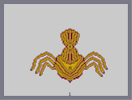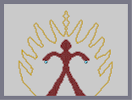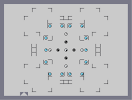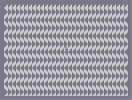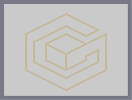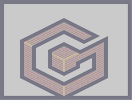Minoin of the Aphocylips ULTRA NINJA! Drone Tribal Dance Triangular My Avatar My Avatar(Tile Set Version)